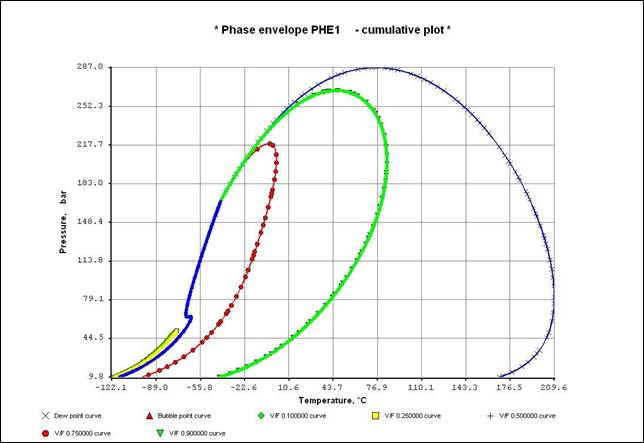VESSEL DEPRESSURIZATION

FIRE SCENARIO

View:               Process Flow Diagram

# Problem Description

This example presents the analysis of the depressurization of a plant equipment in case of fire. The equipment considered is a section of a christmas-tree operated on an off-shore platform.

The simulation process flow diagram contains:

·         a phase envelope calculation for the analysis of the pvt behavior of the fluid

·         a DEPRESS unit operation to simulate the behavior of the christmas-tree content

·         a pipeline to verify the blow-down line for the initial relief

The composition of the fluid (stream S1) is detailed on the next table.

 Component molar flowrate kmol/h 1 WATER 1.305028 2 CARBON DIOXIDE 0.66088 3 NITROGEN 0.22587 4 METHANE 67.08345 5 ETHANE 5.71368 6 PROPANE 2.409282 7 ISOBUTANE 0.435009 8 BUTANE 0.828191 9 ISOPENTANE 0.267698 10 PENTANE 0.460106 11 HEXANE 0.610686 12 HEPTANE 0.426644 13 OCTANE 0.711073 14 NONANE 0.619052 15 DECANE 0.309526 16 UNDECANE 0.250967 17 DODECANE 0.234236 18 C13+ 0.81146

The phase envelope of the mixture in shown on Fig. 1

Fig. 1The Christmas-tree is simulated as a horizontal vessel with an internal diameter of 0.196 m and a length of about 15 m.

Vessel weight is set at 3250 kg, to take into account the effect of metal inertia during the heating caused by fire.

Heat model according to API RP521 recommended practice is assumed with a reduction factor of 0.3. Heat flow is also scaled with respect to the liquid volume content.

Pressure safety valve relief pressure is set at 257.9 bar.

Due to the very critical results the calculation time step used is quite low, it is set at 0.5 seconds.

Pressure and temperature variation with respect to time is shown on the next figure 2.

Fig. 2An unexpected and potentially dangerous pressure increase is experienced after an initial pressure reduction. This may be related to the fact that the fluid appears to be quite near supercritical conditions with a relevant low heat of vaporization.

Results should be carefully analyzed by process and mechanical engineers to verify whether the maximum pressure is compatible with the design pressure of the equipment.

# Generated Keyword Input File

<XPSIM>  ...generated by XpsimWin v.1.07 ...

*

RUN ID='FIRE 01' CUSTOMER=STAFF PROJECT='BLOW DOWN'

DESC  BLOW-DOWN

DESC COLD CASE VERIFICATION

DIMENSION INPUT SI PRES=BAR

Petroleum Data

HYPO ID=C13+ NAME=C13+ NBP=248 DENS=816.5 MW=199.5

System Data

CHEMCOMP H2O / CO2 / N2 / C1 / C2 / C3 / IC4 / NC4 / IC5 / NC5 / NC6 +

/ NC7 / NC8 / NC9 / NC10 / NC11 / NC12

THERMSET UID=M1

METHODS K=SRK HS=LK CP=LK D=LK IV=LK TRAN=IDEAL SURT=IDEAL

REGION PHASE=VAP

WOPT K=2

Flowsheet Data

<DISABLE>

EQUI 2PHASE

</DISABLE>

STREAM=S1 TEMP=15 PRES=257.9 RATE(W)=2080 XBASIS=M

COMP H2O:1.56 / CO2:0.79 / N2:0.27 / C1:80.19 / C2:6.83 / C3:2.88 / +

IC4:0.52 / NC4:0.99 / IC5:0.32 / NC5:0.55 / NC6:0.73 / NC7:0.51 / +

NC8:0.85 / NC9:0.74 / NC10:0.37 / NC11:0.30 / NC12:0.28 / C13+:0.97

PHASENV IN S1 UID=PHE1

CALC PMAX=400 VF=0.1,0.25,0.5,0.75,0.9

DEPRESS IN S1 OUT S5 UID=DEP1

VESSEL TYPE=HORZ DIAM(MT)=0.196 LENGTH=14.92 WEIGHT=3250 CP=0.5

CALC MODEL=1 PHASE=VAP PRELIEF=257.9 PFINAL=6 TFINAL(MIN)=20 +

TSTEP(SEC)=0.5 PBACK=2 NOSTOP TCAL POUT=2

PSV MODEL=5 CONST=0.075 KB=1 KC=1 KD=1

PRODUCT IRELIEF=S5

HEAT MODEL=5 AREA=9.184 QFAC=0.3

PRINT PLOT TINT(SEC)=10

PSD IN S5 OUT S7 UID=PSD1

PARA CALC=1 METHOD=MXFLOW PSET=260 PBACK=3

SIZE DEVICE=VALVE AREA(CM2)=1 KB=1 KC=1 KD=1

SETSTR UID=ST1 STR=S5A

COPY STR=S5

PIPELINE IN S7 OUT S9 UID=PL1

PARA PHASE=MIX COORD=CURV STEP(MT)=1

CORRELAT SET=MECH2F

AMBIENT AIR TEMP(CENT)=15 V=1 UID=AIR1

POINT DIST(MT)=0 ELEV(MT)=0 DIAM(MM)=73 AMB=AIR1 K(W/MC)=55,,,, +

THICK(MM)=15,,,,

POINT DIST=12 ELEV=0

PRINT TRACE STEP PROP

END